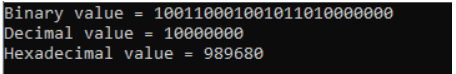# Type Casting in C

## Type Casting in C Language

In this article, I am going to discuss Type Casting in C Language with examples. Please read our previous article, where we discussed the Data Types in C Language. As part of this article, you will learn what is Type Casting in C, and why do we need Typecasting in C Language with examples.

##### Why do we need Type Casting in C?

If both data types are the same type then the return value will be the same. If both data types are different then among those two bigger ones will be the return type. When we are working with integral value with the combination of float then always return value will be float type. Let us understand this with an example.

```#include <stdio.h>
int main()
{
int  i = 10000;
long int l;
float f;
i = 32767 + 1;
l = 32767 + 1;
f = 32767.0f + 1;
printf("%d %ld %f", i, l, f );
return 0;
}```

Output: 32768 32768 32768.000000

But what if you want to store a bigger value like a float in a smaller variable like int. Then in such cases, we need typecasting.

##### What is Type Casting in C Language?

It is a concept of converting one data type values into another data type. Typecasting is performing by using the cast operator. The compiler will automatically change one type of data into another if it makes sense. For instance, if you assign an integer value to a floating-point variable, the compiler will convert the int to float. Casting allows you to make this type of conversion explicit, or to force it when it wouldn’t normally happen.

Type conversion in c can be classified into the following two types:

• Implicit Type Conversion
• Explicit Type Conversion
###### Syntax: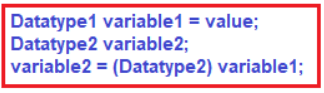###### Example to Understand Type Casting in C Language:
```#include <stdio.h>
int main ()
{
float f = 10.25;
int i;
i = (int) f;
printf ("%d %f", i, f);
return 0;
}```
###### Output: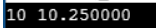In the above C program, we are trying to store the floating value in an integer variable. So, we typecast it before storing it into the integer variable.

Note: It is best practice to convert lower data types to higher data types to avoid data loss. Data will be truncated when a higher data type is converted to lower. For example, if the float is converted to int, data that is present after the decimal point will be lost.

##### What is the cast operator in C?

Cast operator is a data type present in between parenthesis after the equal (=) operator and before the source variable.

##### Implicit Type Conversion or Implicit Type Casting in C:

When the type of conversion is performed automatically by the compiler without the programmer’s intervention, such type of conversion is known as implicit type conversion or type promotion.

It is the concept of converting lower data type values into higher data types. Implicit type casting is under the control of the compiler. So a programmer no needs to be considered the implicit type casting process. Let us see an example for a better understanding.

```#include <stdio.h>
int main ()
{
int i = 12345;
long int l;
l = (long)i; // Implicit casting from int to long int
printf("%d %d", i,l);
return 0;
}```

Output: 12345 12345

##### Explicit Type Conversion or Explicit Type Casting in C:

The type conversion performed by the programmer by posing the data type of the expression of a specific type is known as explicit type conversion. The explicit type conversion is also known as typecasting.

It is a process of converting a higher data type value into a lower data type. Whenever the explicit type casting has occurred mandatory handling or else data overflow will occur. The typecasting in c is done in the following form:

(data_type) expression; where, data_type is any valid c data type, and expression may be constant, variable, or expression. Let us see a program for understanding this concept

```#include <stdio.h>
int main ()
{
double d = 12345.6789;
int l;
l = (int)d; //Explicit casting from double to int
printf("%d %lf", l, d);
return 0;
}```

Output: 12345 12345.678900

##### Rules for Type Casting in C:

The following rules have to be followed while converting the expression from one type to another to avoid the loss of information:

1. All integer types are to be converted to float.
2. All float types are to be converted to double.
3. All character types are to be converted to an integer.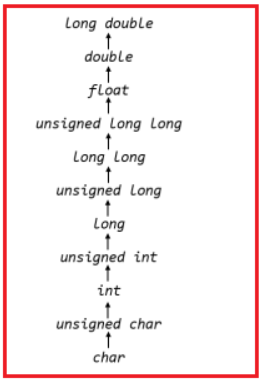Example:
int x=7, y=5;
float z;
z=x/y; /*Here the value of z is 1*/

If we want to get the exact value of 7/5 then we need explicit casting from int to float:

Example:
int x=7, y=5;
float z;
z = (float)x/(float)y; /*Here the value of z is 1.4*/

##### Inbuilt TypeCast Functions in C Language

There are many inbuilt typecasting functions available in C language which performs data type conversion from one type to another.

• atof() Converts a string to float
• atoi() Converts a string to int
• atol() Converts a string to long
• itoa() Converts int to string
• ltoa() Converts long to string
##### atof() function in C:

The atof() function in the C language converts string data type to float data type. The “stdlib.h” header file supports all the typecasting functions in the C language.
Syntax: double atof (const char* string);

##### Example to understand the atof() function in C:
```#include <stdio.h>
#include <stdlib.h>
int main()
{
char a = "3.14";
float pi = atof(a);
printf("Value of pi = %f\n", pi);
return 0;
}```

Output: Value of pi = 3.140000

##### atoi() function in C:

The atoi() function in C language converts string data type to int data type.
Syntax: int atoi (const char * str);

##### Example to understand the C atoi() function:
```#include <stdio.h>
#include <stdlib.h>
int main()
{
char a = "100";
int value = atoi(a);
printf("Value = %d\n", value);
return 0;
}```

Output: Value = 100

##### atol() function in C:

The atol() function in C language converts string data type to long data type.
Syntax: long int atol ( const char * str );

##### Example to understand atol function in C:
```#include <stdio.h>
#include <stdlib.h>
int main()
{
char a = "100000000000";
long value = atol(a);
printf("Value = %ld\n", value);
return 0;
}```

Output: Value = 1215752192

##### itoa() function in C:

The itoa () function in the C language converts int data type to string data type.
Syntax: char *  itoa ( int value, char * str, int base );

##### Example to understand itoa function in C:
```#include <stdio.h>
#include <stdlib.h>
#include <string.h>
int main()
{
int a=54325;
char buffer;
itoa(a,buffer,2);   // here 2 means binary
printf("Binary value = %s\n", buffer);
itoa(a,buffer,10);  // here 10 means decimal
printf("Decimal value = %s\n", buffer);
itoa(a,buffer,16);   // here 16 means Hexadecimal
return 0;
}```
###### Output: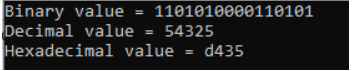Note: “stdlib.h” header file supports all the typecasting functions in C language. But, it is a nonstandard function.

##### ltoa() function in C:

The ltoa() function in the C language converts long data type to string data type.
Syntax: char *ltoa(long N, char *str, int base);

##### Example to understand C ltoa function:
```#include <stdio.h>
#include <stdlib.h>
#include <string.h>
int main()
{
long  a=10000000;
char buffer;
ltoa(a,buffer,2);   // here 2 means binary
printf("Binary value = %s\n", buffer);
ltoa(a,buffer,10);   // here 10 means decimal
printf("Decimal value = %s\n", buffer);
ltoa(a,buffer,16);   // here 16 means Hexadecimal